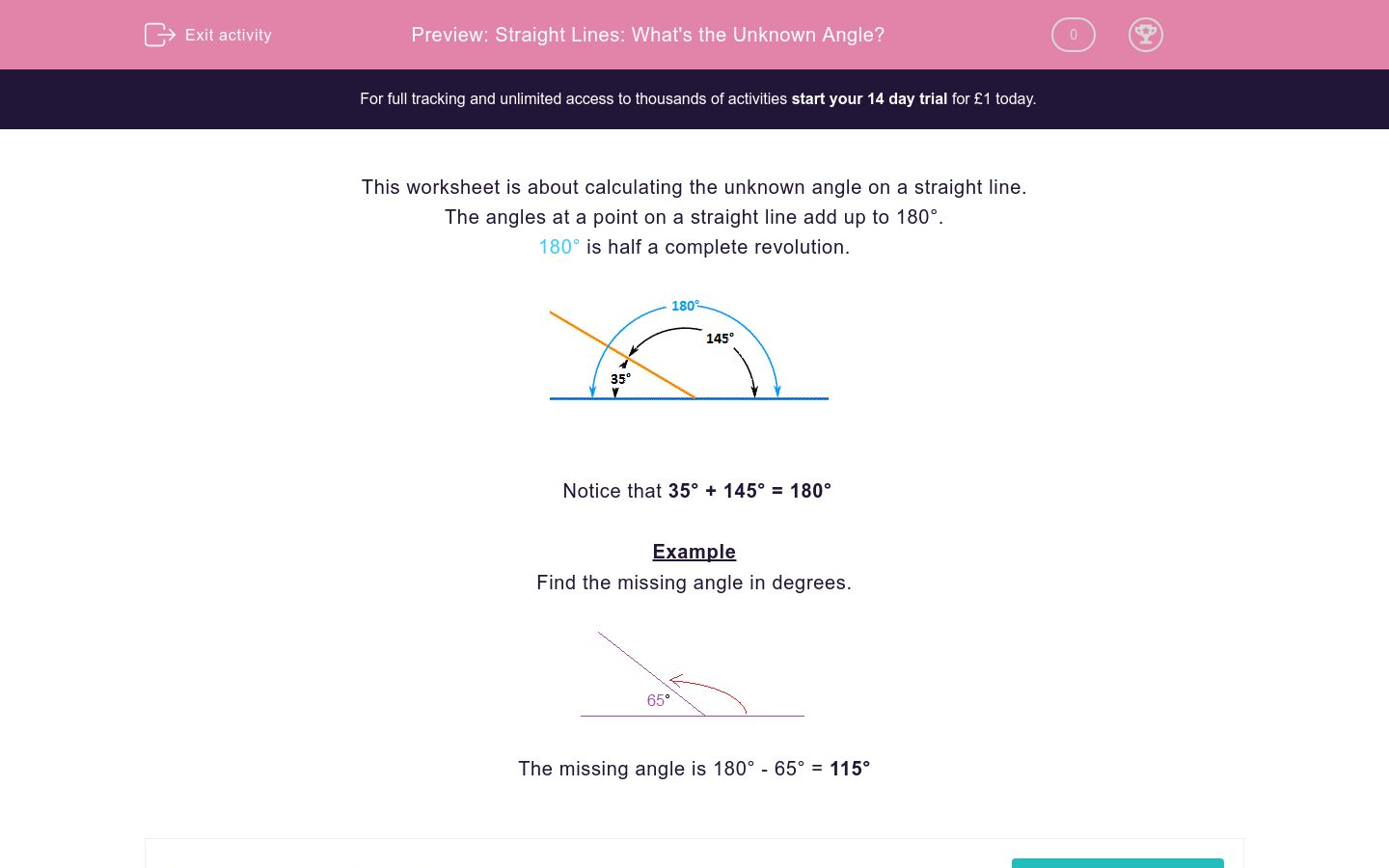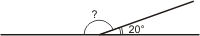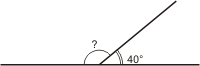# Straight Lines: What's the Unknown Angle?

In this worksheet, students calculate the unknown angle on a straight line.Key stage:  KS 2

Curriculum topic:   Maths and Numerical Reasoning

Curriculum subtopic:   2D Shapes: Circles, Angles and Bearings

Difficulty level:### QUESTION 1 of 10

This worksheet is about calculating the unknown angle on a straight line.

The angles at a point on a straight line add up to 180°.

180° is half a complete revolution.Notice that 35° + 145° = 180°

Example

Find the missing angle in degrees.The missing angle is 180° - 65° = 115°

Calculate the unknown angle in degrees.  (Just write the number)Calculate the unknown angle in degrees.  (Just write the number)Calculate the unknown angle in degrees.  (Just write the number)Calculate the unknown angle in degrees.  (Just write the number)Calculate the unknown angle in degrees.  (Just write the number)• Question 1

Calculate the unknown angle in degrees.  (Just write the number)160
• Question 2

Calculate the unknown angle in degrees.  (Just write the number)40
• Question 3

Calculate the unknown angle in degrees.  (Just write the number)60
• Question 4

Calculate the unknown angle in degrees.  (Just write the number)140
• Question 5

Calculate the unknown angle in degrees.  (Just write the number)120
---- OR ----

Sign up for a £1 trial so you can track and measure your child's progress on this activity.

### What is EdPlace?

We're your National Curriculum aligned online education content provider helping each child succeed in English, maths and science from year 1 to GCSE. With an EdPlace account you’ll be able to track and measure progress, helping each child achieve their best. We build confidence and attainment by personalising each child’s learning at a level that suits them.

Get started